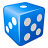# Conditional Numbering points/polygons in ArcGIS 2010

3127
4
08-18-2015 10:06 PMNew Contributor

In Excel, I can do:

In cell B2 =IF(A2=A1, B1+1,1)

Data in A1:A20

A     B

1     1

1     =IF(A2=A1, B1+1,1)

1

2

2

2

2

3

3

...

How can I do in ArcGIS Attribute Table using field calculator?

I use the AutoIncreament() ... but it work according to the OBJECT_ID and that is not CONDITIONAL...

Khong

4 RepliesOccasional Contributor III

I am not sure about accessing previous row values in the Field Calculator but you could do this as a python script - something like this:

```import arcpy

table = "C:/Temp/Data.gdb/Test"
fields = ('A', 'B')
prevA = 0
B = 0

with arcpy.da.UpdateCursor(table, fields) as cursor:
for row in cursor:
# increment or reset B value
if (row == prevA):
B += 1
else:
B = 1
row = B
cursor.updateRow(row)
# set previous A value
prevA = row```New Contributor

I tried it but not working.

The auto increment sorted by OBJECT_ID is okay but no condition!

Pre-Logic Script Code:

rec=0

def autoIncrement():

global rec

pStart = 1 #adjust start value, if req'd

pInterval = 2 #adjust interval value, if req'd

if (rec == 0):

rec = pStart

else:

rec = rec + pInterval

return rec

Expression:

autoIncrement()byMVP Legendary Contributor

If it is a cumulative sum based upon another columns just use this field calculator expression

```# cumulative sum
total = 0
def cumsum(in_field):
global total
total+=in_field

with cumsum(field to sum in here) as in the example below.

Perform a cumulative sum on the Group field and place the result in the Cumu_Sum fieldNew Contributor

I tried it but not working.

The auto increment sorted by OBJECT_ID is okay but no condition!

Pre-Logic Script Code:

rec=0

def autoIncrement():

global rec

pStart = 1 #adjust start value, if req'd

pInterval = 2 #adjust interval value, if req'd

if (rec == 0):

rec = pStart

else:

rec = rec + pInterval

return rec

Expression:

autoIncrement()